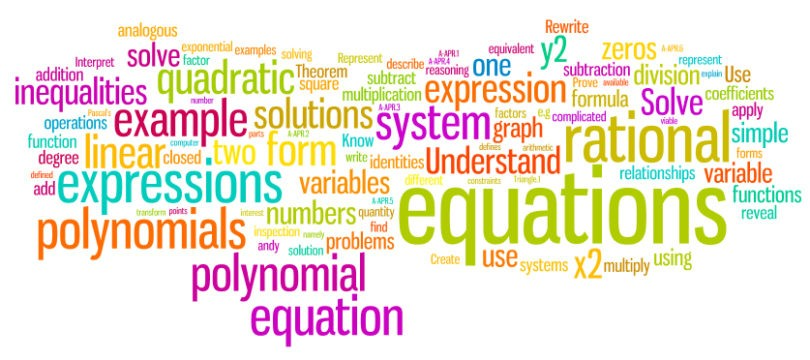# Set Theory question bank### Set Theory Problems with answers:

Ques. If two sets A and B are having 99 elements in common, then the number of elements common to each of the sets A x B and B x A are
(a) 299
(b) 992
(c) 100
(d) 18
Ans. (b)

Related: Digestive system multiple choice

Ques. The set of intelligent students in a class is
(a) A null set
(b) A singleton set
(c) A finite set
(d) Not a well defined collection
Ans. (d)

Ques. Of the members of three athletic teams in a school 21 are in the cricket team, 26 are in the hockey team and 29 are in the football team. Among them, 14 play hockey and cricket, 15 play hockey and football, and 12 play football and cricket. Eight play all the three games. The total number of members in the three athletic teams is
(a) 43
(b) 76
(c) 49
(d) None of these
Ans. (a)

Ques. Which of the following is the empty set
(a) {x : x is a real number and x2 – 1 = 0}
(b) {x : x  is a real number and x2 + 1 = 0}
(c) {x : x is a real number and x2 – 9 = 0
(d) {x : x is a real number and x2 = x + 2
Ans. (b)

Ques. If a set A has n  elements, then the total number of subsets of A  is
(a) n
(b) n2
(c) 2n
(d) 2n
Ans. (c)

Related: Thermodynamics quiz with answers

Ques. If (1, 3), (2, 5) and (3, 3) are three elements of A x B and the total number of elements in A x B is 6, then the remaining elements of A x B are
(a) (1, 5); (2, 3); (3, 5)
(b) (5, 1); (3, 2); (5, 3)
(c) (1, 5); (2, 3); (5, 3)
(d) None of these
Ans. (a)

Ques. A class has 175 students. The following data shows the number of students obtaining one or more subjects. Mathematics 100, Physics 70, Chemistry 40; Mathematics and Physics 30, Mathematics and Chemistry 28, Physics and Chemistry 23; Mathematics, Physics and Chemistry 18. How many students have offered Mathematics alone
(a) 35
(b) 48
(c) 60
(d) 22
Ans. (c)

Related: Probability quiz

Ques. The number of proper subsets of the set {1, 2, 3} is
(a) 8
(b) 7
(c) 6
(d) 5
Ans. (c)

Ques. In a town of 10,000 families it was found that 40% family buy newspaper A, 20% buy newspaper B and 10% families buy newspaper C, 5% families buy A and B, 3% buy B and C and 4% buy A and C. If 2% families buy all the three newspapers, then number of families which buy A only is
(a) 3100
(b) 3300
(c) 2900
(d) 1400
Ans. (b)

Related: questions on Structure of Atom

Ques. In a city 20 percent of the population travels by car, 50 percent travels by bus and 10 percent travels by both car and bus. Then persons travelling by car or bus is
(a) 80 percent
(b) 40 percent
(c) 60 percent
(d) 70 percent
Ans. (c)

Ques. Let S = {0, 1, 5, 4, 7}. Then the total number of subsets of S is
(a) 64
(b) 32
(c) 40
(d) 20
Ans. (b)

Related: p block elements questions

Ques. A set contains 2n + 1 elements. The number of subsets of this set containing more than n elements is equal to
(a) 2n – 1
(b) 2n
(c) 2n + 1
(d) 22n
Ans. (d)

Ques. 20 teachers of a school either teach mathematics or physics. 12 of them teach mathematics while 4 teach both the subjects. Then the number of teachers teaching physics only is
(a) 12
(b) 8
(c) 16
(d) None of these
Ans. (a)

Related: questions on Complex Numbers

Ques. In a battle 70% of the combatants lost one eye, 80% an ear, 75% an arm, 85% a leg, x% lost all the four limbs. The minimum value of x is
(a) 10
(b) 12
(c) 15
(d) None of these
Ans. (a)

Ques. If A = {1, 2, 4}, B = {2, 4, 5}, C = {2, 5}, then (AB) x (BC) is
(a) {(1, 2), (1, 5), (2, 5)}
(b) {(1, 4)}
(c) (1, 4)
(d) None of these
Ans. (b)

Ques. If n(A) = 4, n(B) = 3, n(A x B x C) = 24, then n(C) =
(a) 288
(b) 1
(c) 12
(d) 2
Ans. (d)

Related: Nomenclature test online

Ques. In a class of 30 pupils, 12 take needle work, 16 take physics and 18 take history. If all the 30 students take at least one subject and no one takes all three then the number of pupils taking 2 subjects is
(a) 16
(b) 6
(c) 8
(d) 20
Ans. (a)

### What is Set Theory?

Set theory is a fundamental concept in mathematics. Its definitions include numbers, functions and functionals, as well as geometric and topological concepts. It is often referred to as the Foundations of Mathematics, since any question of mathematical provability can be reduced to its formal derivation.

### About the author

#### Yash Bansal

Yash is co-founder of Examsegg. Yash loves to solve questions on reasoning and mathematics. He taught himself how to blend different techniques to get accurate solution in lesser time.

### 1 Comment

• Apporva says:

Hello